# 自然语言处理 09：词汇语义学

## 墨尔本大学 COMP90042 课程笔记

Posted by YEY on April 1, 2020

# Lecture 09 词汇语义学

## 1. 词汇语义学

### 1.1 例子：情感分析

• 词袋模型，KNN 分类器。训练数据：
• $\textit{“This is a good movie.”}\quad \to \quad$ 正面 😊
• $\textit{“This is a great movie.”}\quad \to \quad$ 正面 😊
• $\textit{“This is a terrible film.”}\quad \to \quad$ 负面 🙁
• $\textit{“This is a wonderful film.”}\quad \to \quad$ ?

KNN 分类器会将这个句子分类为负面情感 🙁。

• 有两个问题：
• 模型并不知道 “$\textit{movie}$” 和 “$\textit{film}$” 是同义词。由于 “$\textit{film}$” 仅在负面样本中出现过，所以，模型学习到的是：这是一个负面单词。
• “$\textit{wonderful}$” 这个单词在词汇表中并没有出现过（OOV, Out-Of-Vocabulary）
• 直接比较单词并不是一种很好的方法。我们应当如何保证我们是在比较单词的 含义 呢？
• 解决方案：通过一个 词汇数据库（lexical database）来显式地加入这些信息。

### 1.2 单词语义学

• 词汇语义学（Lexical semantics）
• 单词之间的含义如何相互联系。
• 手动构建资源：词汇表 (lexicons)、同义词词典 (thesauri)、本体论 (ontologies) 等。

我们可以用文本来描述单词的含义，我们也可以观察不同单词之间是如何相互联系的。例如：单词 “$\textit{film}$” 和 “$\textit{movie}$” 实际上是 同义词（synonym），所以，假如我们不知道 “$\textit{film}$” 的意思，但是我们知道 “$\textit{movie}$” 的意思，并且假如我们还知道两者是同义词关系的话，我们就可以知道单词 “$\textit{film}$” 的意思。我们将看到如何通过手工构建这样的词汇数据库，这些同义词词典或者本体论捕获了单词含义之间的联系。

• 分布语义学（Distributional semantics）
• 文本中的单词之间如何互相关联。
• 从语料库中自动创建资源。

我们也可以用另一种方式完成同样的事情。我们的任务仍然是捕获单词的含义，但是相比雇佣语言学家来手工构建词汇数据库，我们可以尝试从语料库中直接学习单词含义。我们尝试利用机器学习或者语料库的一些统计学方法来观察单词之间是如何互相关联的，而不是从语言学专家那里直接得到相关信息。

### 1.3 单词的含义是什么？

• 物理或社交世界中的被引用的对象
• 但通常在文本分析中没有用

回忆你小时候尝试学习一个新单词的场景，对于人类而言，单词的含义包含了对于物理世界的引用。例如：当你学习 “$\textit{dog}$（狗）” 这个单词时，你会问自己，什么是 “$\textit{dog}$”？你不会仅通过文本或者口头描述来学习这个单词，而是通过观察真实世界中的狗来认识这个单词，这其中涉及到的信息不止包含语言学，而且还包括狗的叫声、气味等其他信息，所有这些信息共同构成了 “$\textit{dog}$” 这个单词的含义。但是这些其他的信息通常在文本分析中并没有太大作用，并且我们也不容易对其进行表示。

• 单词的字典定义
• 但是字典定义需要是 循环的（circular）
• 仅在已经理解含义的情况下才有用

因此，我们希望寻找一种其他方法来学习单词的含义：通过查词典学习单词含义。但是，我们会发现词典定义通常带有循环性质，我们用一些其他单词来解释目标单词。下面是一个例子：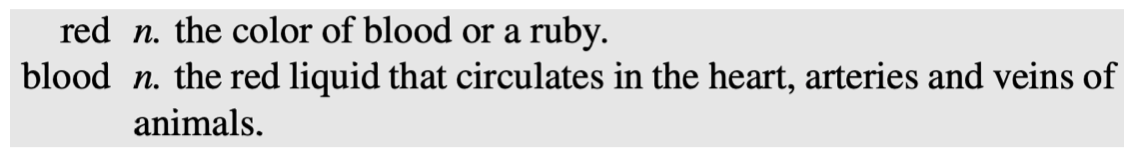可以看到，在定义 “$\textit{red}$（红色）” 这个单词时，我们将其描述为 “$\textit{blood}$（血液）” 的颜色；然后在定义 “$\textit{blood}$（血液）” 这个单词时，我们将其描述为心脏中的一种 “$\textit{red}$（红色）” 液体。所以，我们用 “$\textit{blood}$” 定义 “$\textit{red}$”，然后又用 “$\textit{red}$” 定义 “$\textit{blood}$”。如果我们本身不知道这两个单词的含义，那么我们无法从定义中获得词义。但是，字典定义仍然是非常有用的，因为当我们通过字典学习一个新的单词时，我们通常已经具有了一定的词汇背景，例如当我们学习一门新的语言时，字典可以提供一些非常有用的信息。

• 目标单词与其他单词的关系
• 也是循环的，但更实用

另一种学习词义的方法是查看目标单词和其他单词的关系。同样，这种方法也涉及到循环性的问题，但是，当我们需要结合上下文使用某个单词时，这种方法非常有用，就像之前 “$\textit{film}$” 和 “$\textit{movie}$” 的例子。所以，单词之间的关系是另一种非常好的表征词义的方式。

### 1.4 词义

$\textbf{mouse}^{\mathbf 1}$: $\dots \text{a } \textit{mouse } \text{controlling a computer system in 1968.}$
$\textbf{mouse}^{\mathbf 2}$: $\dots \text{a quite animal like a } \textit{mouse.}$
$\textbf{bank}^{\mathbf 1}$: $\dots \text{a } \textit{bank } \text{can hold the investments in a custodial account}\dots$
$\textbf{bank}^{\mathbf 2}$: $\dots \text{as agriculture burgeons on the east } \textit{bank} \text{, the river}\dots$

### 1.5 单词注解

• $\textit{Bank}$ :
• 接受存款并将资金用于贷款活动的金融机构
• 倾斜的土地（尤其是水体旁的斜坡）

### 1.6 通过关系定义单词含义

• 同义（Synonymy）：几乎相同的含义。
• 两个词含义相同，则互为 同义词 (synonym)
• $\textit{vomit}\,$ vs. $\textit{throw up}$
• $\textit{big}\,$ vs. $\textit{large}$
• 反义（Antonymy）：相反的含义。
• 两个词含义相反，则互为 反义词 (antonym)
• $\textit{long}\,$ vs. $\textit{short}$
• $\textit{big}\,$ vs. $\textit{little}$
• 上位关系（Hypernymy）：is-a 的关系。
• 前者为 下位词 (hyponym)，表示后者的一个更加具体的实例，例如 $cat$。
• 后者为 上位词 (hypernym)，表示比前者更宽泛的一个类别，例如 $animal$。
• $\textit{cat } \text{is an } \textit{animal}$
• $\textit{mango } \text{is a } \textit{fruit}$
• 部分整体关系（Meronymy）：part-whole 的关系。
• 前者为 部件词 (meronym)，表示后者的一部分，例如 $leg$。
• 后者为 整体词 (holonym)，表示包含前者的一个整体，例如 $table$。
• $\textit{leg } \text{is part of a } \textit{chair}$
• $\textit{wheel } \text{is part of a } \textit{car}$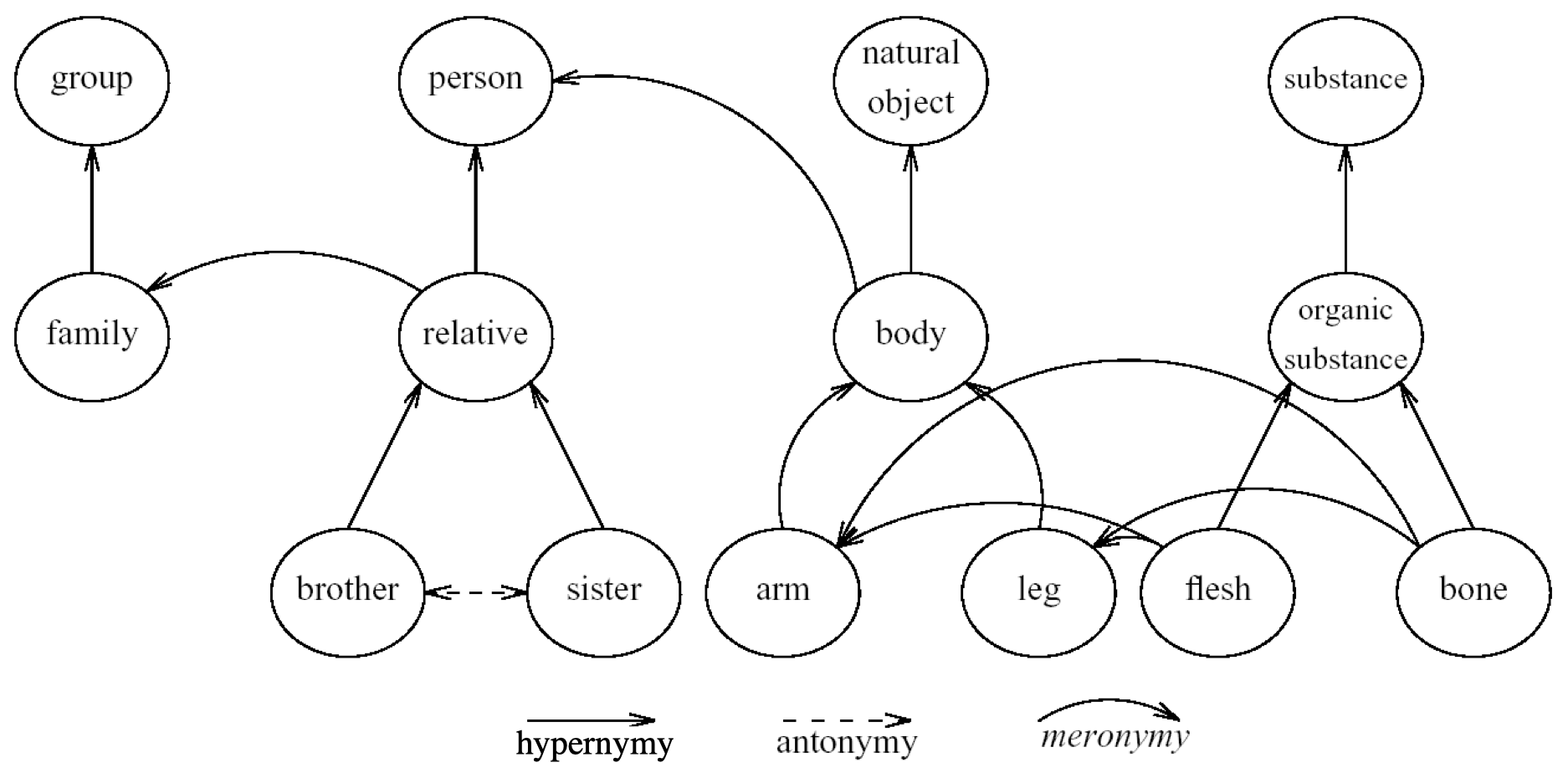### 1.7 WordNet

• 一个词汇关系的数据库
• 平均来看：一个名词具有 1.23 个词义（senses）；一个动词具有 2.16 个词义。
• WordNet 囊括了大部分的主流语言：
• 英语版本可以免费获取（通过 NLTK）

WordNet 例子： 名词 “$\textit{bass}$” 在 WordNet 中有 8 个词义（senses）。

1. $\text{bass}^1 - (\text{the lowest part of the musical range})$
2. $\text{bass}^2, \text{bass part}^1 - (\text{the lowest part in polyphonic music})$
3. $\text{bass}^3, \text{basso}^1 - (\text{an adult male singer with the lowest voice})$
4. $\text{sea bass}^1, \text{bass}^4 - (\text{the lean flesh of a saltwater fish of the family Serranidae})$
5. $\text{freshwater bass}^1, \text{bass}^5 - \,(\text{any of various North American freshwater fish with}$
$\qquad \qquad \qquad \qquad \quad \,\text{lean flesh (especially of the genus Micropterus)})$
6. $\text{bass}^6, \text{bass voice}^1, \text{basso}^2 - (\text{the lowest male adult singing voice})$
7. $\text{bass}^7 - (\text{the member with the lowest range of a family of musical instruments})$
8. $\text{bass}^8 - \, (\text{nontechnical name for any of numerous edible marine and freshwater}$
$\qquad \quad \text{spiny-finned fishes})$

### 1.8 同义词集（Synsets）

• WordNet 中的结点并不是单词（words）或者词目（lemmas），而是词义（senses）。
• 它们被表示为同义词（synonyms）的集合，被称为 同义词集（synsets）

• 单词 $\textit{bass}$ 的同义词集：
• $\{\text{bass}^1, \text{deep}^6\}$
• $\{\text{bass}^6, \text{bass voice}^1, \text{basso}^2\}$
• 另一个同义词集：
• $\{\text{chump}^1, \text{fool}^2, \text{gull}^1, \text{mark}^9, \text{pasty}^1, \text{fall guy}^1, \text{sucker}^1, \text{soft touch}^1, \text{mug}^2\}$
• 注解（Gloss）：易受骗且易于利用的人。
1
2
3
4
5
6
7
8
9
10
11
12
13
14
15
# 查看某个单词所有的同义词集
>>> nltk.corpus.wordnet.synsets(‘bank’)
[Synset('bank.n.01'), Synset('depository_financial_institution.n.01'), Synset('bank.n.03'),
Synset('bank.n.04'), Synset('bank.n.05'), Synset('bank.n.06'), Synset('bank.n.07'),
Synset('savings_bank.n.02'), Synset('bank.n.09'), Synset('bank.n.10'), Synset('bank.v.01'),
Synset('bank.v.02'), Synset('bank.v.03'), Synset('bank.v.04'), Synset('bank.v.05'),
Synset('deposit.v.02'), Synset('bank.v.07'), Synset('trust.v.01')]

# 查看某个单词的某个同义词集的定义
>>> nltk.corpus.wordnet.synsets(‘bank’).definition()
u'sloping land (especially the slope beside a body of water)‘

# 查看某个单词的某个同义词集中的所有相关词目
>>> nltk.corpus.wordnet.synsets(‘bank’).lemma_names()
[u'depository_financial_institution', u'bank', u'banking_concern', u'banking_company']


### 1.9 WordNet 中的词汇关系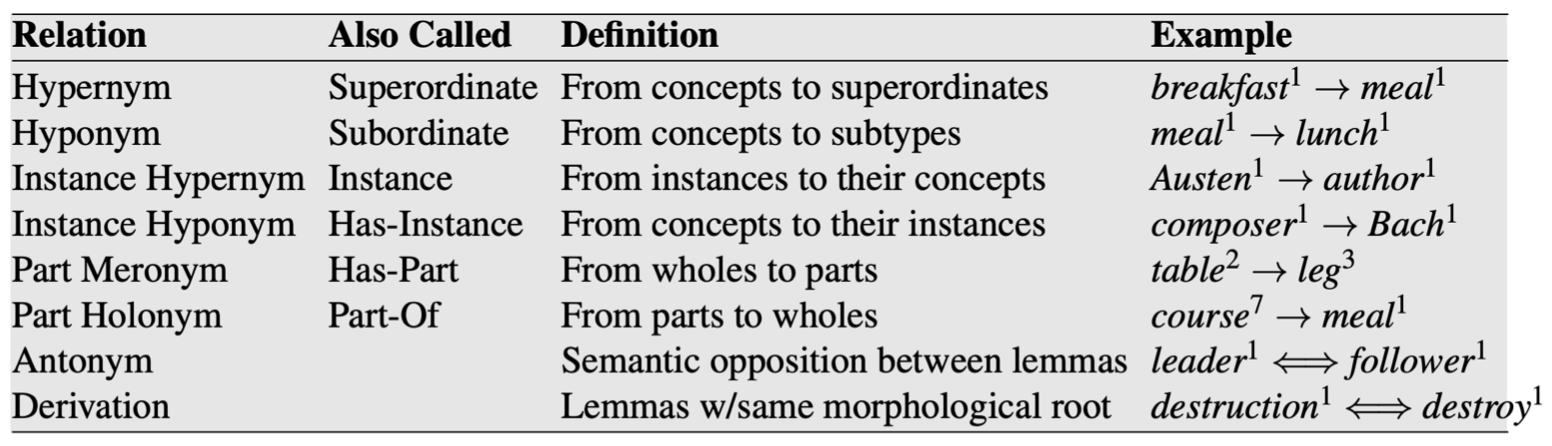### 1.10 上位关系链（Hypernymy Chain）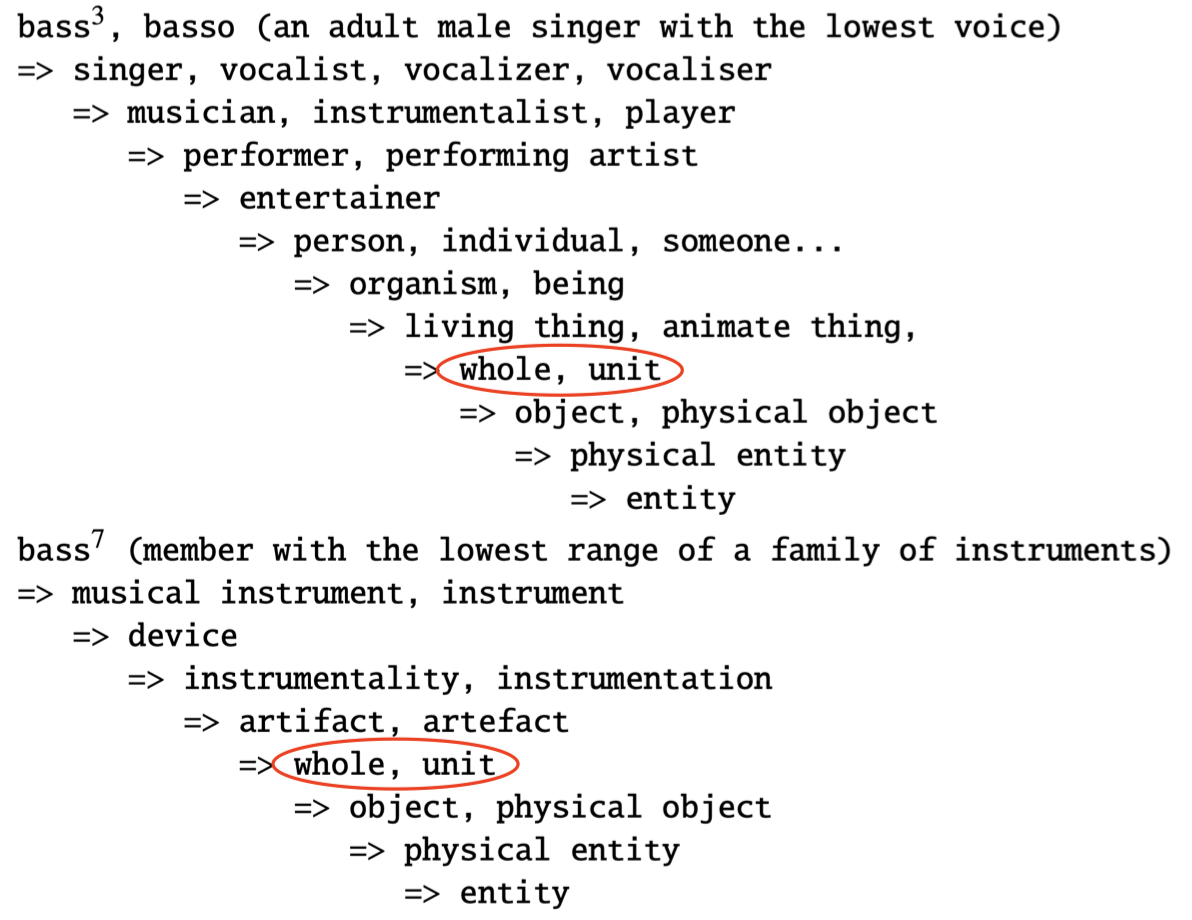## 2. 单词相似度

### 2.1 单词相似度（Word Similarity）

• 同义（Synonymy）：$\textit{film}\,$ vs. $\textit{movie}$
我们已经见过了同义关系，并且我们可以从词汇关系数据库中捕获到这种同义关系。
• 但是，如果是 $\textit{show}\,$ vs. $\textit{film}$ 或者 $\textit{opera}\,$ vs. $\textit{film}$ 呢？
这些单词的含义并非完全相同，但是非常接近。
• 与同义词（二元关系，binary relation）不同，单词相似度是一个 谱（spectrum）
如果我们仅仅采用二元关系作为标准，我们将无法囊括上述关系（相似/相关但不相同），因此，我们希望构建一种谱来衡量单词之间的相似度，例如：$\textit{opera}$ 和 $\textit{film}$ 之间的相似度要高于 $\textit{flower}$ 和 $\textit{film}$ 之间的相似度。
• 我们可以词汇数据库（例如：WordNet）或者同义词词典（thesaurus）来评估单词相似度。

### 2.2 基于路径的单词相似度

• 给定 WordNet，基于路径长度寻找相似度。
• 路径长度 $\text{pathlen}(c_1,c_2)=1+$ 词义 $c_1$ 和 $c_2$ 之间的最短路径的边长。
• 两个词义（senses）之间的相似度：
• $\text{simpath}(c_1,c_2)=\dfrac{1}{\text{pathlen}(c_1,c_2)}$
• 两个单词（words）之间的相似度：
• $\text{wordsim}(w_1,w_2)=\max \limits_{c_1\in \text{ senses}(w_1), c_2\in \text{ senses}(w_2)}\text{simpath}(c_1,c_2)$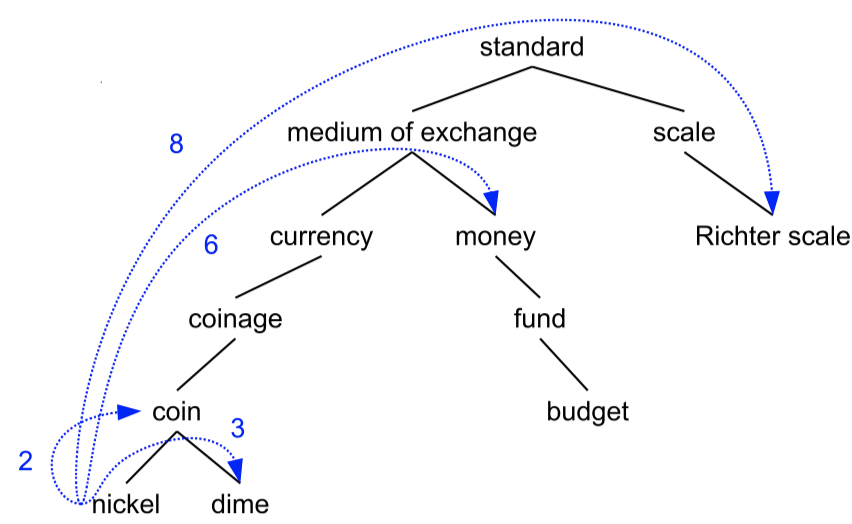• $\text{simpath}(\textit{nickel},\textit{coin})=1/2=0.5$
• $\text{simpath}(\textit{nickel},\textit{currency})=1/4=0.25$
• $\text{simpath}(\textit{nickel},\textit{money})=1/6=0.17$
• $\text{simpath}(\textit{nickel},\textit{Richter scale})=1/8=0.13$

### 2.3 超越路径长度

• 问题：每条边在实际语义距离上的差异非常大
• 在靠近顶部层级处，同样一条边的语义跳跃要比靠近底层时更大。
• 因此，单纯依靠路径长度并不能区分这种差异，因为对于每条边，其长度总是为 $1$。
• 解决方案 1：增加深度信息（Wu & Palmer）
• 使用路径查找 最低公共归类（lowest common subsumer，LCS）
• 利用深度进行比较
$\text{simwup}(c_1,c_2)=\dfrac{2\times\text{depth}(LCS(c_1,c_2))}{\text{depth}(c_1)+\text{depth}(c_2)}$• $\text{simwup}(\textit{nickel},\textit{money})=\dfrac{2\times 2}{3+6}=0.44$
• $\text{simwup}(\textit{nickel},\textit{Richter scale})=\dfrac{2\times 1}{3+6}=0.22$

### 2.4 抽象结点

• 层次结构中位于较高的节点是非常抽象/一般的。
• 我们如何能够使得通过非常抽象的节点连接的单词之间的相似度大大降低。

### 2.5 概念概率（Concept Probability）

$P(c)=\dfrac{\sum_{w\in \text{words}(c)}\mathrm{count}(w)}{N}$
• $P(c)$：从语料库中随机选择的一个单词是概念 $c$ 的一个实例的概率。
• $\text{words}(c)$：$c$ 的所有子结点的单词的集合。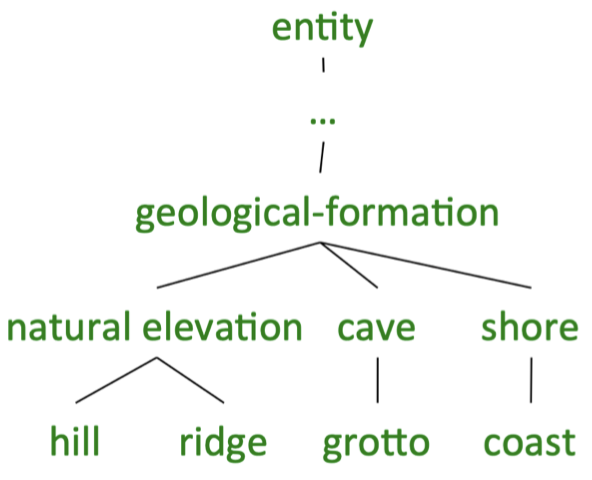$\text{words}(\textit{geological-formation}) = \{\textit{hill}, \textit{ridge}, \textit{grotto}, \textit{coast}, \textit{natural elevation}, \textit{cave}, \textit{shore}\}$ $\text{words}(\textit{natural elevation}) = \{\textit{hill}, \textit{ridge}\}$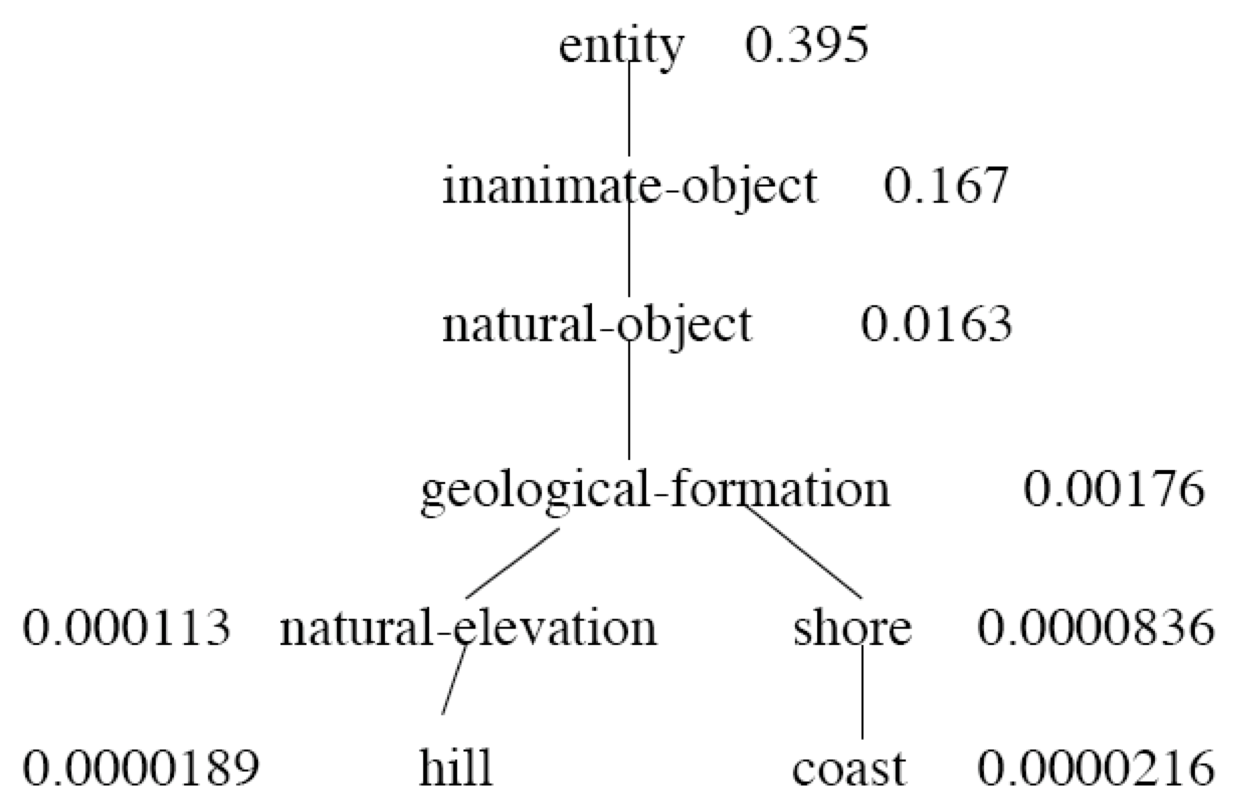### 2.6 基于信息量的相似度

\begin{align} IC(c) &= -\log P(c) \\\\ \mathrm{simlin}(c_1,c_2) &= \dfrac{2\times IC(LCS(c_1,c_2))}{IC(c_1)+IC(c_2)} \end{align}\begin{align} \mathrm{simlin}(c_1,c_2) &= \dfrac{2\times -\log P(\textit{geological-formation})}{-\log P(\textit{hill})-\log P(\textit{coast})} \\ &= \dfrac{-2\log 0.00176}{-\log 0.0000189-\log 0.0000216}\\ &= 0.587 \end{align}

### 2.7 回顾之前情感分析的例子

• $\textit{“This is a good movie.”}\quad \to \quad$ 正面 😊
• $\textit{“This is a wonderful film.”}\quad \to \quad$ ?
• 如果我们的分类器是基于单词相似度（例如：KNN），使用 WordNet 路径比较单词效果较好。
• 但是，如果我们希望将词义（sense）作为一般特征表示，那么我们该如何使用其他分类器？
• 解决方案：将文本中的单词（words）显式地映射到 WordNet 中的词义（senses）。

### 2.8 词义消歧

• 任务：为句子中的单词选择正确的词义。
• Baseline：
• 假设最流行的词义
• 良好的 词义消歧 (Word Sense Disambiguation, WSD) 在很多 NLP 任务中都有应用潜力。
• 在实践中经常被忽略，因为良好的 WSD 是非常困难的。
• 在研究领域中非常活跃。

### 2.9 有监督 WSD

• 采用标准的机器学习分类器
• 特征向量通常是目标周围的单词和句法
• 但是上下文也可能带有 歧义（ambiguous）
• 上下文窗口应该设置为多大？（通常很小）
• 需要带 词义标记（sense-tagged）的语料库
• 例如 SENSEVAL 和 SEMCOR（NLTK 中提供）
• 创建过程非常耗时

### 2.10 较少监督的方法

Lesk：选择 WordNet 中的字典注解（dictionary gloss）与上下文重叠最大的词义（sense）。

$\textit{The } \boldsymbol{bank} \textit{ can guarantee deposits will eventually cover future tuition costs because it invests}$ $\textit{in adjustable-rate mortgage securities.}$

• $\mathbf{bank^1}$：有 2 个重叠的非停用词，$\textit{deposits}$ 和 $\textit{mortgage}$
• $\mathbf{bank^2}$：没有重叠的非停用词。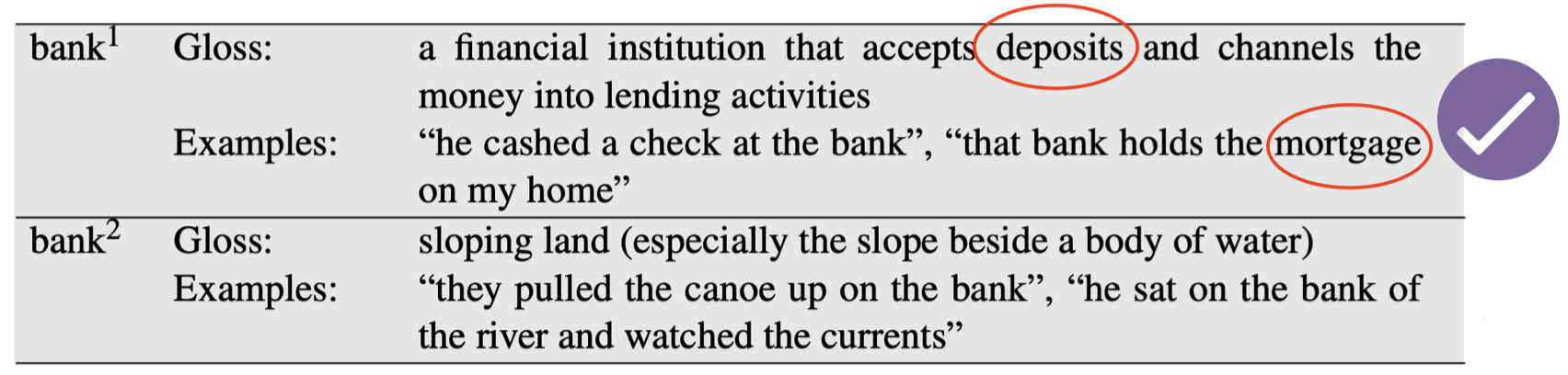### 2.11 其他数据库：FrameNet

• 基于 框架语义（frame semantics）
• $\textit{Mary bought a car from John.}$
玛丽从约翰那里买了辆车
• $\textit{John sold a car to Mary.}$
约翰把车卖给了玛丽。
• 相同的情况（语义框架），只是视角不同。
• 一个 框架（frames）的词汇数据库，通常是典型情况
• 例如 “$\textit{commerce_buy}$”、“$\textit{apply_heat}$”

### 2.12 FrameNet

• 包含引发框架的 词汇单元（lexical units）的列表。
• 例如：$\textit{cook, fry, bake, boil}$ 等等
• 语义角色（semantic roles）框架元素（frame elements）的列表。
• 例如：$\textit{“the cook”, “the food”, “the container”, “the instrument”}$
• 框架之间的 语义关系（Semantic relationships）
• 例如：$\textit{“apply_heat”}$ 是 $\textit{“absorb_heat”}$ 的成因，并且被 $“cooking_creation”$ 使用。

### 2.13 进入语料库

• 手动标记的词汇资源是文本分析的一个重要起点。
• 但是，许多现代工作都试图在没有人工干预的情况下，直接从语料库中获取语义信息。
• 分布式语义学（Distributional semantics）

## 3. 扩展阅读

• JM3 Ch 19.1-19.3, 19.4.1, 19.5.1本作品采用知识共享署名-非商业性使用-相同方式共享 4.0 国际许可协议进行许可。 欢迎转载，并请注明来自：YEY 的博客 同时保持文章内容的完整和以上声明信息！